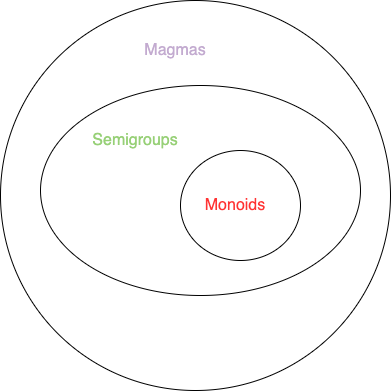# 理解函数式编程中的函数组合--Monoids(二)

## Monoids

### 可聚合的事物

1 + 2 = 3


"a" + "b" = "ab"


• 有两个事物
• 两个事物能够通过一种组合方式将他们组合起来
• 得到的事物跟之前的类型是一致的

type Money = {
amount: number
};

const a: Money = { amount: 10.2 }
const b: Money = { amount: 2.8 }
const c: Money = { amount: a.amount + b.amount }


[0, 2, 3, 4].reduce((acc, val) => acc + val);
["a", "b", "c", "d"].reduce((acc, val) => acc + val);


MapReduce大家应该都不陌生，为什么叫Map? 因为需要将数据转化为Monoids, 为什么要Reduce? 因为需要聚合数据。

### 结合律

(1 + 2) + 3 = 1 + (2 + 3) = 6


(1 * 2) * 3 = 1 * (2 * 3) = 6


(1 - 2) - 3 != 1 - (2 - 3)
(1 % 2) % 3 != 1 % (2 % 3)


### Identity元素

1 + 0 = 1
"a" + "" = "a"


10 * 1 = 10


const a = [1, 2, 3]
const b: number[] = []
const c = [...a, ...b]

expect(c).toEqual(a);


const a: number[] = [];
const result = a.reduce((acc, val) => acc + val);


const result = a.reduce((acc, val) => acc + val, 0);


### Monoids定律

• 定律1 (Closure): 两个事物合并后总能得到相同类型的事物。
• 定律2 (Associativity): 当组合一组事物时，组合的顺序不会影响结果(不是交换律的那种顺序）。
• 定律3 (Identity element): 有一个0元素，任何事物跟0元素合并之后的结果不变。
用数学家的话说，凡是符合上面三个定律的事物被称之为Monoids。符合定律1的叫做Magma, 同时符合定律1和定律2的称之为Semigroups。interface Monoid<A> {
readonly concat: (x: A, y: A) => A
}


concat(x, concat(y, z)) = concat(concat(x, y), z)


class Monoid m where
mempty :: m
mappend :: m -> m -> m
mconcat :: [m] -> m
mconcat = foldr mappend mempty


• mempty代表Identty 元素
• mappend代表一个函数，接受两个相同类型的参数，然后返回一个类型也一样的值，可以理解为二元操作符
• mconcat是一个函数，接受一组monoid值，然后聚合为一个值。它拥有一个默认实现，使用mappend操作符和mempty作为默认值，来fold一个列表

## 函数也可以是Monoids

type func = <T1, T2>(x: T1) => T2


T1 -> T2


instance Monoid b => Monoid (a -> b)


type func = <T>(x: T) => T


## Monoid实战

type OrderLine = {
id: number,
name: string,
quality: number,
total: number
}


const total = orderLines.reduce((acc, val) => acc.total + val.total)


OrderLine -> OrderLine -> number


OrderLine -> OrderLine -> OrderLine


const addTwoOrderLines = (line1: OrderLine, line2: OrderLine): OrderLine => (
{
name: "total",
quality: line1.quality + line2.quality,
total: line1.total + line2.total
}
)